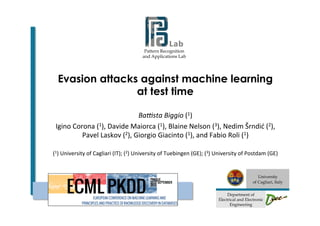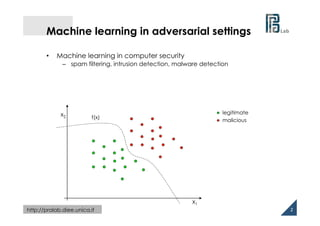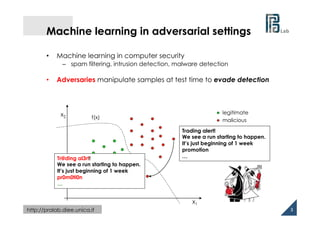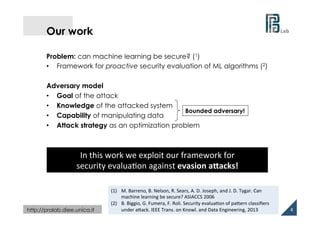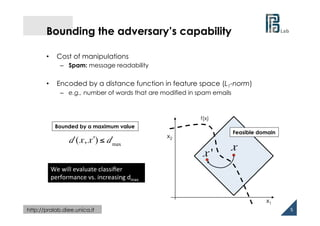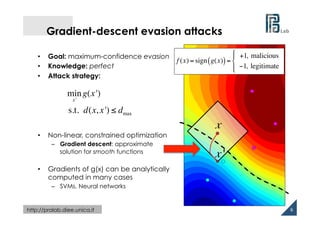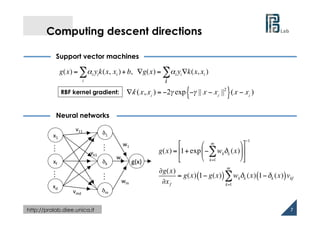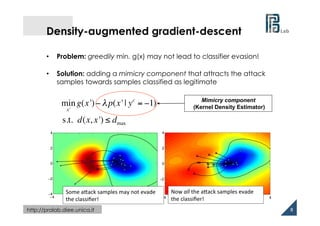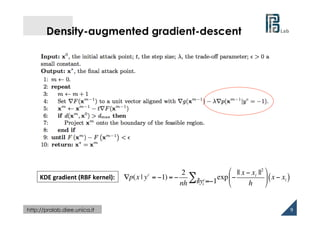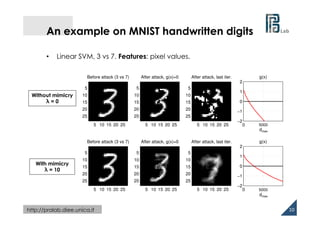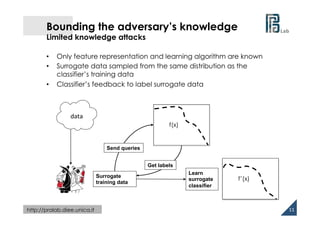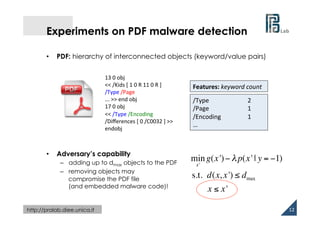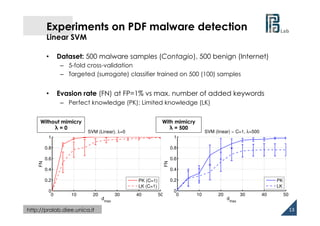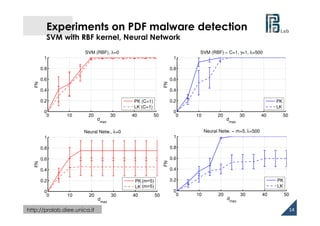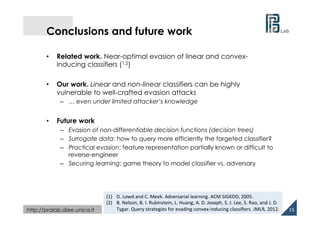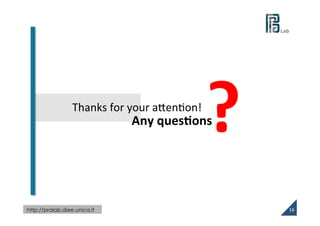1 of 16

### Battista Biggio @ ECML PKDD 2013 - Evasion attacks against machine learning at test time

1. Pattern Recognition and Applications Lab                                   University of Cagliari, Italy   Department of Electrical and Electronic Engineering Evasion attacks against machine learning at test time Ba#sta  Biggio  (1)   Igino  Corona  (1),  Davide  Maiorca  (1),  Blaine  Nelson  (3),  Nedim  Šrndić  (2),   Pavel  Laskov  (2),  Giorgio  Giacinto  (1),  and  Fabio  Roli  (1)     (1)  University  of  Cagliari  (IT);  (2)  University  of  Tuebingen  (GE);  (3)  University  of  Postdam  (GE)
2.   http://pralab.diee.unica.it Machine learning in adversarial settings •  Machine learning in computer security –  spam filtering, intrusion detection, malware detection legitimate malicious x1   x2   f(x) 2
3.   http://pralab.diee.unica.it Machine learning in adversarial settings •  Machine learning in computer security –  spam filtering, intrusion detection, malware detection •  Adversaries manipulate samples at test time to evade detection legitimate malicious x1   x2   f(x) 3   Trading alert! We see a run starting to happen. It’s just beginning of 1 week promotion …Tr@ding al3rt! We see a run starting to happen. It’s just beginning of 1 week pr0m0ti0n …
4.   http://pralab.diee.unica.it Our work Problem: can machine learning be secure? (1) •  Framework for proactive security evaluation of ML algorithms (2) Adversary model •  Goal of the attack •  Knowledge of the attacked system •  Capability of manipulating data •  Attack strategy as an optimization problem 4   Bounded adversary! (1)  M.  Barreno,  B.  Nelson,  R.  Sears,  A.  D.  Joseph,  and  J.  D.  Tygar.  Can   machine  learning  be  secure?  ASIACCS  2006   (2)  B.  Biggio,  G.  Fumera,  F.  Roli.  Security  evaluaVon  of  paWern  classiﬁers   under  aWack.  IEEE  Trans.  on  Knowl.  and  Data  Engineering,  2013   In  this  work  we  exploit  our  framework  for   security  evaluaVon  against  evasion  a)acks!
5.   http://pralab.diee.unica.it Bounding the adversary’s capability •  Cost of manipulations –  Spam: message readability •  Encoded by a distance function in feature space (L1-norm) –  e.g., number of words that are modified in spam emails 5   d (x, !x ) ≤ dmax x2   x1   f(x) Bounded by a maximum value x Feasible domain x ' We  will  evaluate  classiﬁer   performance  vs.  increasing  dmax
6.   http://pralab.diee.unica.it Gradient-descent evasion attacks •  Goal: maximum-confidence evasion •  Knowledge: perfect •  Attack strategy: •  Non-linear, constrained optimization –  Gradient descent: approximate solution for smooth functions •  Gradients of g(x) can be analytically computed in many cases –  SVMs, Neural networks 6   −2−1.5−1−0.500.51 x f (x) = sign g(x)( )= +1, malicious −1, legitimate " # \$ %\$ min x' g(x') s.t. d(x, x') ≤ dmax x '
7.   http://pralab.diee.unica.it Computing descent directions Support vector machines Neural networks 7   x1   xd   δ1   δk   δm   xf   g(x)   w1   wk   wm   v11   vmd   vk1   …… …… g(x) = αi yik(x, i ∑ xi )+ b, ∇g(x) = αi yi∇k(x, xi ) i ∑ g(x) = 1+exp − wkδk (x) k=1 m ∑ # \$ % & ' ( ) * + , - . −1 ∂g(x) ∂xf = g(x) 1− g(x)( ) wkδk (x) 1−δk (x)( )vkf k=1 m ∑ RBF kernel gradient: ∇k (x,xi ) = −2γ exp −γ || x − xi ||2 { }(x − xi )
8.   http://pralab.diee.unica.it g(x) − λ p(x|yc=−1), λ=0 −4 −3 −2 −1 0 1 2 3 4 −4 −2 0 2 4 −1 −0.5 0 0.5 1 •  Problem: greedily min. g(x) may not lead to classifier evasion! •  Solution: adding a mimicry component that attracts the attack samples towards samples classified as legitimate Density-augmented gradient-descent Mimicry component (Kernel Density Estimator) 8   g(x) − λ p(x|yc=−1), λ=20 −4 −3 −2 −1 0 1 2 3 4 −4 −2 0 2 4 −4.5 −4 −3.5 −3 −2.5 −2 −1.5 −1 Now  all  the  aWack  samples  evade   the  classiﬁer!   Some  aWack  samples  may  not  evade   the  classiﬁer!     min x' g(x')− λp(x' | yc = −1) s.t. d(x, x') ≤ dmax
9.   http://pralab.diee.unica.it Density-augmented gradient-descent 9   ∇p(x | yc = −1) = − 2 nh exp − || x − xi ||2 h # \$ % & ' ( x − xi( )i|yi c=−1∑KDE  gradient  (RBF  kernel):
10.   http://pralab.diee.unica.it An example on MNIST handwritten digits 10   •  Linear SVM, 3 vs 7. Features: pixel values. Before attack (3 vs 7) 5 10 15 20 25 5 10 15 20 25 After attack, g(x)=0 5 10 15 20 25 5 10 15 20 25 After attack, last iter. 5 10 15 20 25 5 10 15 20 25 0 500 −2 −1 0 1 2 g(x) number of iterations Without mimicry λ = 0 dmax 5000 Before attack (3 vs 7) 5 10 15 20 25 5 10 15 20 25 After attack, g(x)=0 5 10 15 20 25 5 10 15 20 25 After attack, last iter. 5 10 15 20 25 5 10 15 20 25 0 500 −2 −1 0 1 2 g(x) number of iterations With mimicry λ = 10 dmax 5000
11.   http://pralab.diee.unica.it Bounding the adversary’s knowledge Limited knowledge attacks •  Only feature representation and learning algorithm are known •  Surrogate data sampled from the same distribution as the classifier’s training data •  Classifier’s feedback to label surrogate data 11   PD(X,Y)data   Surrogate training data f(x) Send queries Get labels Learn surrogate classifier f’(x)
12.   http://pralab.diee.unica.it Experiments on PDF malware detection •  PDF: hierarchy of interconnected objects (keyword/value pairs) •  Adversary’s capability –  adding up to dmax objects to the PDF –  removing objects may compromise the PDF file (and embedded malware code)! 12   /Type    2   /Page    1   /Encoding  1   …   13  0  obj   <<  /Kids  [  1  0  R  11  0  R  ]   /Type  /Page   ...  >>  end  obj   17  0  obj   <<  /Type  /Encoding   /Diﬀerences  [  0  /C0032  ]  >>   endobj     Features:  keyword  count   min x' g(x')− λp(x' | y = −1) s.t. d(x, x') ≤ dmax x ≤ x'
13.   http://pralab.diee.unica.it 0 10 20 30 40 50 0 0.2 0.4 0.6 0.8 1 dmax FN SVM (Linear), λ=0 PK (C=1) LK (C=1) Experiments on PDF malware detection Linear SVM 13   0 10 20 30 40 50 0 0.2 0.4 0.6 0.8 1 SVM (linear) − C=1, λ=500 dmax FN PK LK •  Dataset: 500 malware samples (Contagio), 500 benign (Internet) –  5-fold cross-validation –  Targeted (surrogate) classifier trained on 500 (100) samples •  Evasion rate (FN) at FP=1% vs max. number of added keywords –  Perfect knowledge (PK); Limited knowledge (LK) Without mimicry λ = 0 With mimicry λ = 500
14.   http://pralab.diee.unica.it Experiments on PDF malware detection SVM with RBF kernel, Neural Network 14   0 10 20 30 40 50 0 0.2 0.4 0.6 0.8 1 Neural Netw. − m=5,λ=500 dmax FN PK LK 0 10 20 30 40 50 0 0.2 0.4 0.6 0.8 1 SVM (RBF) − C=1, γ=1, λ=500 dmax FN PK LK 0 10 20 30 40 50 0 0.2 0.4 0.6 0.8 1 dmax FN SVM (RBF), λ=0 PK (C=1) LK (C=1) 0 10 20 30 40 50 0 0.2 0.4 0.6 0.8 1 dmax FN Neural Netw., λ=0 PK (C=1) LK (C=1) (m=5) (m=5)
15.   http://pralab.diee.unica.it Conclusions and future work •  Related work. Near-optimal evasion of linear and convex- inducing classifiers (1,2) •  Our work. Linear and non-linear classifiers can be highly vulnerable to well-crafted evasion attacks –  … even under limited attacker’s knowledge •  Future work –  Evasion of non-differentiable decision functions (decision trees) –  Surrogate data: how to query more efficiently the targeted classifier? –  Practical evasion: feature representation partially known or difficult to reverse-engineer –  Securing learning: game theory to model classifier vs. adversary 15   (1)  D.  Lowd  and  C.  Meek.  Adversarial  learning.  ACM  SIGKDD,  2005.   (2)  B.  Nelson,  B.  I.  Rubinstein,  L.  Huang,  A.  D.  Joseph,  S.  J.  Lee,  S.  Rao,  and  J.  D.   Tygar.  Query  strategies  for  evading  convex-­‐inducing  classiﬁers.  JMLR,  2012.
16.   http://pralab.diee.unica.it ?   16     Any  ques@ons  Thanks  for  your  aWenVon!